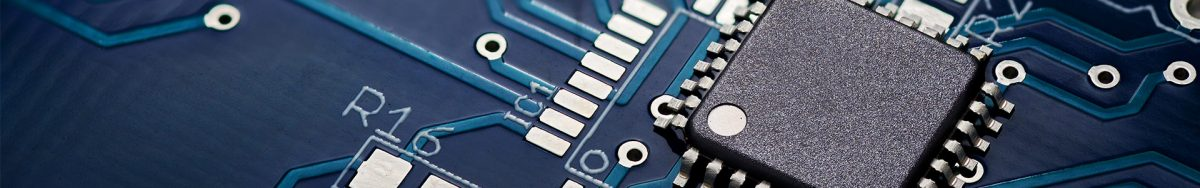# Crystal Equivalent Circuit### The equivalent circuit of a quartz crystal is as follows:The equivalent circuit consists of L1 (motional inductance), and C0(shunt capacitance). All the parameters can be customized through design but are tied together so that a change to one parameter changes other parameters.

L1: The motional inductance of the crystal is determined by the mechanical mass (of quartz) in motion. The lower frequencies (thicker and larger quartz wafers) tend to run at a few henries where higher frequencies (thinner and smaller quartz wafers) tend to run at a few millihenries.

The L1 and C1 are related by Thomson’s formula:

L1= 1/(4 2fC1)

It is preferable to have the customer specify if necessary. Then the L1 will follow the above formula.

C1: The motion capacitance of a crystal is determined by the stiffness of the quartz (which is constant), the area of metallization (electrode size) on the face of the crystal, and the thickness and shape of the wafer. At lower frequencies, the wafer must be shaped (contoured or beveled) to improve the performance of the device. The C1 for fundamental made crystals can range from approximately .005pF to .030pF. As a general rule, if a fundamental design is used on an overtone, the C1 will divide by the square of the overtone (i.e., 3rd overtone will be 1/9 of the fundamental).https://suntsu.com/products/suntsu/frequency-control/crystals/ C0: The shunt capacitance of a crystal is due in part to the thickness of the wafer. This is the measured capacitance while not vibrating. This is the measured capacitance ranges from 1- 7pF. It is not typical due to compatibility with the oscillator circuit.